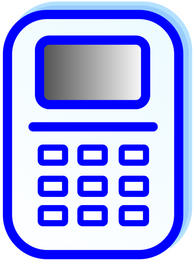## Advanced scientific calculator with 2D / 3D / 4D graphs, imaginary numbers, formulas, matrices, system of equations, integrals & more.## Main window

Main window with math expressions and results in a list. Click any previous result/math expression to edit them.

## Calculator

You can use the calculator for basic and advanced functions. "Trig" button expands to inverse and hiperbolic trigonometric functions.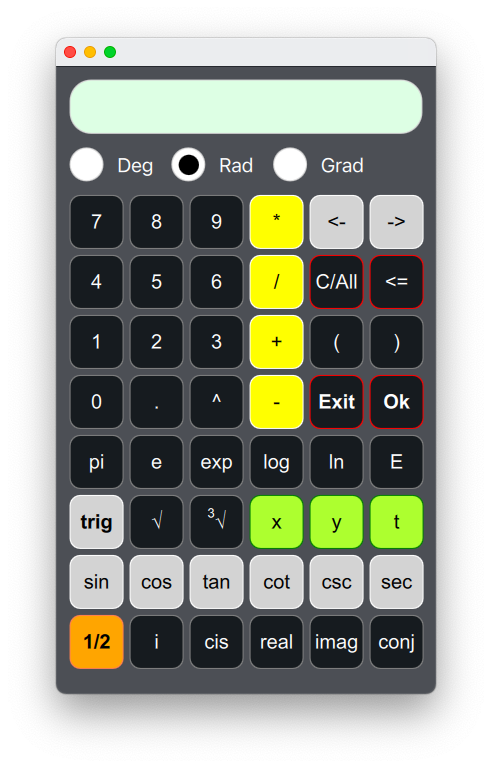## 2D / 3D / 4D graphs

View impressive 3D or animated 4D graphs (with a time variable). You can add many graphs, rotate and zoom them.

## Formulas

Easily add any formula and solve it to any given variable.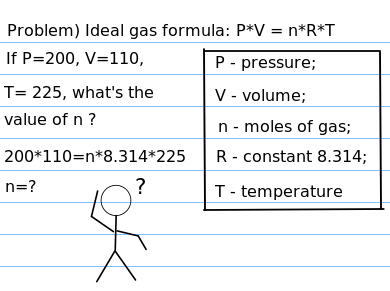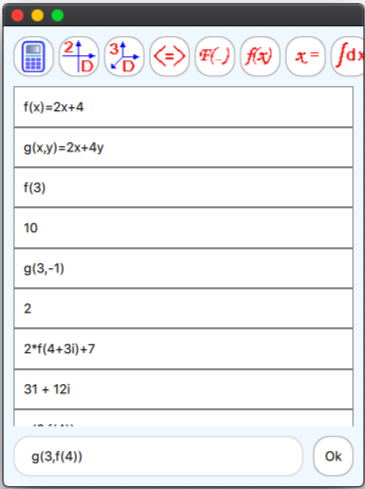## Math functions

Add math functions and solve them nice and easy. You can encapsulate functions inside functions, use imaginary numbers as arguments.

## System of equations

Easily use system of equations with imaginary numbers. The image on the right shows five equations, but we tested with hundreds of equations, with very good performance when solving the system.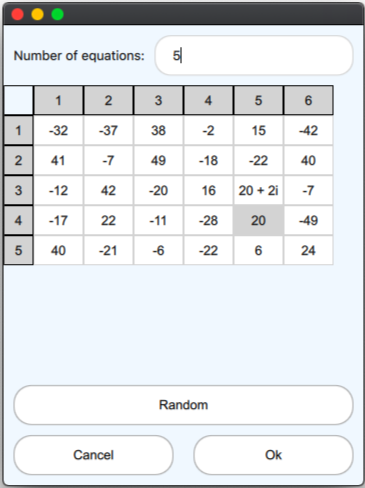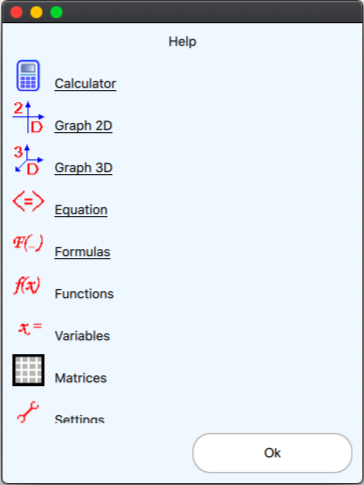Help menu, so you can explore all the features in a very easy way.

## Android Free Version (click bellow):

There are two desktop versions:
-Desktop classic, a widget 90's style
-Desktop modern, a 21 century app style